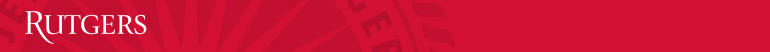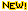﻿ General PhysicsMath & Science Learning Center﻿ Download Notice that homework solutions, Lecture Notes, and exams are all available in PDF format (Adobe Acrobat Reader is required to view and print files).

 Lecture Notes: Solutions to Practice Problems: Practice Exams: Mathematical Concepts Kinematics in one Dimension Kinematics in two Dimensions Newton's Laws of Motion Dynamics of Circular Motion Work and Energy Impulse and Momentum Rotational Kinematics Rotational Dynamics Elasticity and SHO Fluids Temperature and Heat Ideal Gas and Kinetic Theory Thermodynamics Waves and SoundThe principle of Linear SuperpositionChapter 1 Chapter 2 Chapter 3 Chapter 4 Chapter 5 Chapter 6 Chapter 7 Chapter 8 Chapter 9 Chapter 10 Chapter 11 Chapter 12 Chapter 14 Chapter 15 Chapter 16Chapter 17Exam 1 Practice Exam 1Practice Exam 1 SolutionsExam 2 Practice Exam 2Practice Exam 2 SolutionsFinal Practice FinalPractice Final Solutions